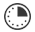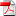# Scale It Up

A. Wills
Multiple Subject Teacher

This lesson will allow students to demonstrate knowledge use of scale.

##Length of Time: 15-25 Minutes

Featured Programs:

### Common Core Alignment

CCSS.MATH.CONTENT.3.MD.B.3 - Draw a scaled picture graph and a scaled bar graph to represent a data set with several categories. Solve one- and two-step "how many more" and "how many less" problems using information presented in scaled bar graphs. For example, draw a bar graph in which each square in the bar graph might represent 5 pets.

## Objectives & Outcomes

Students will recreate a simple picture on a larger scale.

## Materials Needed

Pictures (sample included), colored pencils, printer paper

## Procedure

### Opening to Lesson

• Ask students if they understand scale.
• Explain that if you are printing something on a larger scale it means you are making it bigger, but each part will be in the same area.
• Tell students you will show them a simple way to do scale.

### Body of Lesson

• Give students the sample picture provided.
• Give each student only one of the pictures though several can be printed on the same page.
• Also provide students with a single sheet of printer paper.
• Have students examine the small drawing.
• Now have students fold the paper in half lengthwise and then fold it in half the other way.
• Have students do the same with the larger sheet of paper.
• Explain that they have created scale. What is on the small paper in a particular scale, can be drawn larger on the blank paper in the same square. This will create a larger scale drawing.

### Closing

• Have students put drawings together to show understanding of scale.
• Allow both drawings to be colored if time permits.

## Assessment & Evaluation

The students will accurately recreate a drawing on a larger scale.

## Modification & Differentiation

Special needs students may be given extended time or a simpler drawing.

•Sample Pictures

## Related Lesson Plans

##### Exchanging Time

The lesson is used for students to practice basic time measurement, and understanding the basic units of time.

##### Feed the Gator

Students will compare numbers with three or more digits using visual cues.

##### Fun Fraction Pizza

Students will create a “pizza” from construction paper divided into 8 slices. They will decorate each slice and then exchange slices with classmates and then evaluate the fractions of slices that they have at the end. For example, 1/8 slices of my own pizza, 4/8 or ½ of pizza that was made by a female, 2/8 or ¼ that was made by my buddy.  Note: Students should have already had some lessons about simplification of fractions.

##### Skittles Graph

Students will learn to create a small bar graph using candy for information.